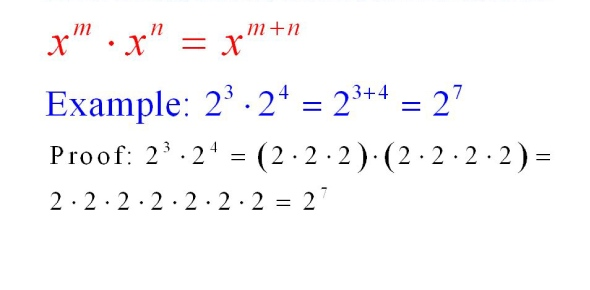Is the following statement true or false? The Multiplication Law of - ProProfs DiscussTopicsMoreProductsMore+ Ask Question

# Is the following statement true or false? The Multiplication Law of Exponents says that for any numbers b, n, and m, bn * bm = bn + m.Change Image    Delete

This question is part of Simplifying Expressions with Exponents
Asked by Acanestra, Last updated: Jul 12, 2020

###Request 0FollowShareAnswer AnonymouslyAnswer LaterCopy Link#### W. Pratt

Want to learn new things and share my knowledge

W. Pratt, Marketing Analyst, MBA, Lincoln

The answer to this question is true considering The Multiplication Law of Exponents says that for any numbers b, n, and m, bn bm = bn + m. Nath can seem intimidating to a lot of people but when you break each equation down, it is just a series of rules that you follow to get the right answer.

Obviously it is not that simplistic and many areas of math have numerous rules and the equation will not work properly if there is any slight mistake in the previous steps. According to this rule, the equation is true.#### N. Kingsley

N. Kingsley, Writer, Columbus

Math consists of rules and laws that enable mathematicians to solve complex problems. According to the law of exponents, the numbers b, n and m, the rule holds true. The rule is bm= bn + m. students need to follow it when solving complex equations in order to arrive at the correct answer.

Failure to follow the basic rule will mislead the student and he will be unable to calculate the correct answer. Likewise there are many other mathematical formulas and laws that require memorization. They are often asked in exams and hold the answer too many of the math problems.The answer is true 100 % sure !!!!

Good luck guys!

Viva mexico putos#### John Smith

John SmithTrueSearch for Google imagesSelect a recommended image
Upload from your computerCancelSearch for Google imagesSelect a recommended image
Upload from your computerCancelSearch for Google imagesSelect a recommended image
Upload from your computerCancel